# 深度学习新应用：在PyTorch中用单个2D图像创建3D模型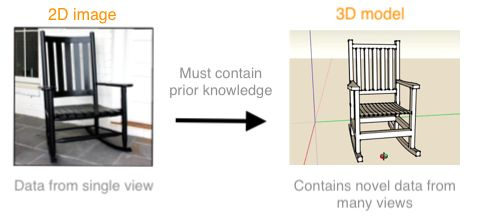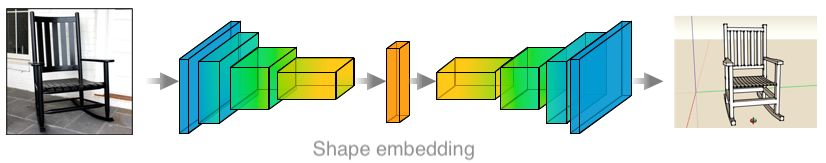CNN 编码深度形状先验知识

3D 数据表征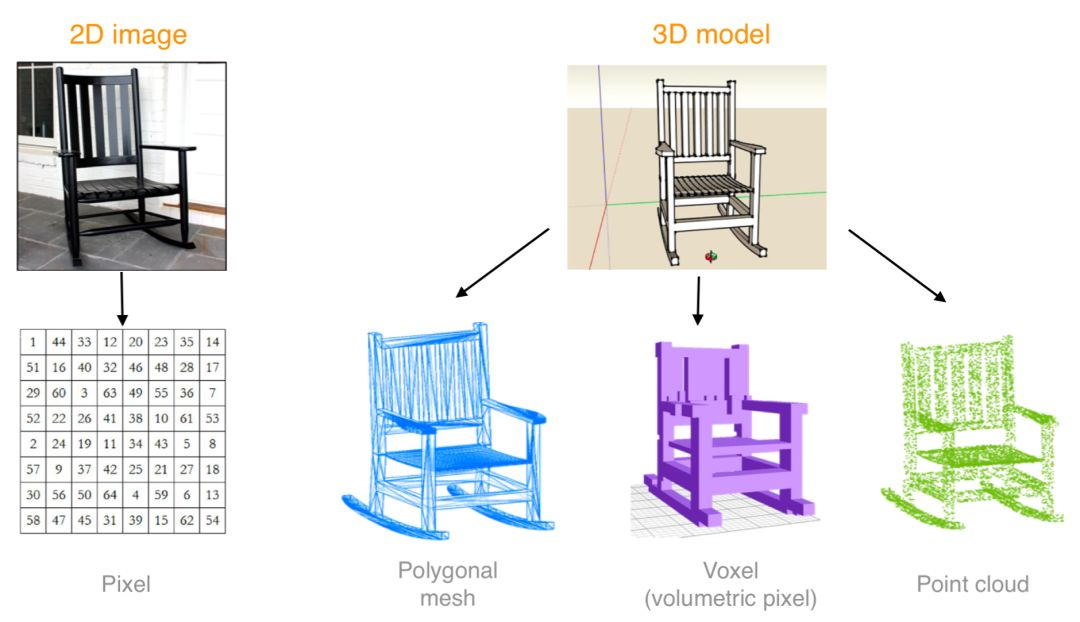3D 数据的不同表征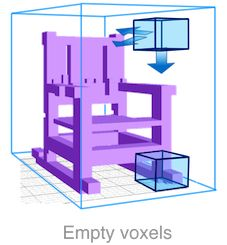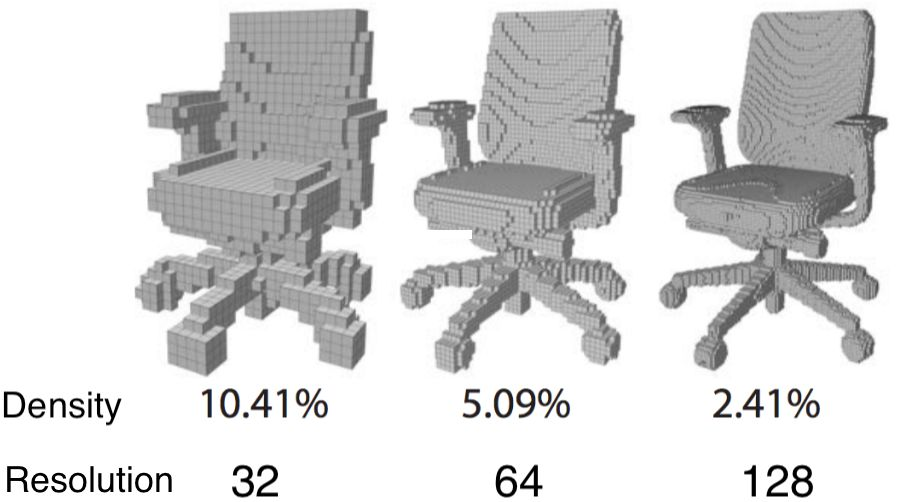• 优势： 从 2D 表征到 3D 表征，可以直接应用 CNN

• 劣势：容易浪费，要在细节和资源（计算力、内存）之间好好权衡。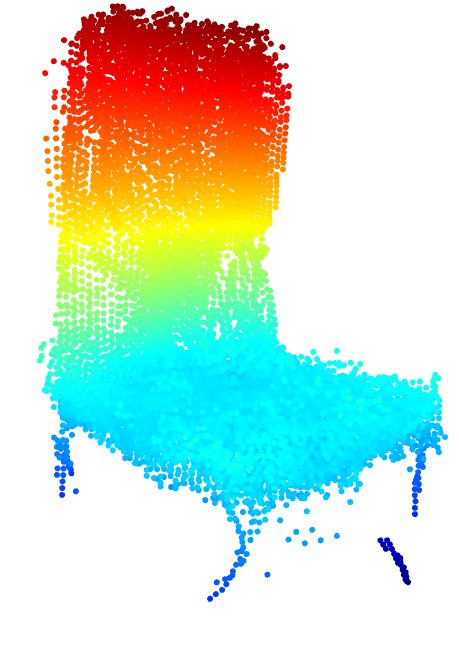• 优势：表征紧凑，重点关注 3D 对象的表面细节。

• 缺点：不能直接应用 CNN。

``````# point_cloud1 and point_cloud2 represent the same 3D structure
# even though they are represented differently in memory
point_cloud1 = [(x1, y1, z1), (x2, y2, z2),..., (xn, yn, zn)]
point_cloud2 = [(x2, y2, z2), (x1, y1, z1),..., (xn, yn, zn)]``````

2D 结构生成器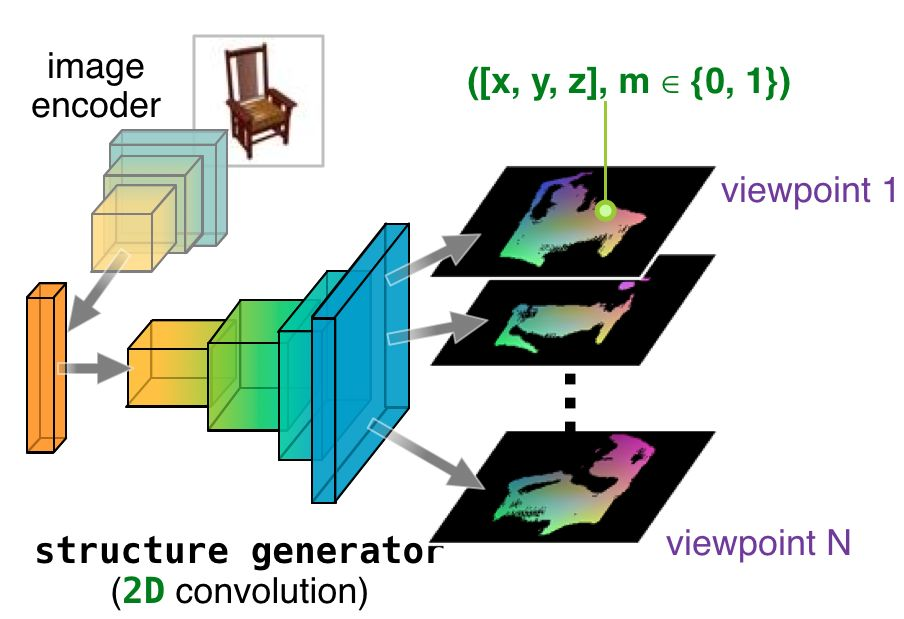• 输入：单个 RGB 图像

• 输出：预先设定视角的 2D 投影

``````#--------- Pytorch pseudo-code for Structure Generator ---------#
class Structure_Generator(nn.Module):
# contains two module in sequence, an encoder and a decoder
def __init__(self):
self.encoder = Encoder()
self.decoder = Decoder()
def forward(self, RGB_image):
# Encoder takes in one RGB image and
# output an encoded deep shape-embedding
shape_embedding = self.encoder(RGB_image)

# Decoder takes the encoded values and output
# multiples 2D projection (XYZ + mask)
XYZ, maskLogit = self.decoder(shape_embedding)

return XYZ, maskLogit``````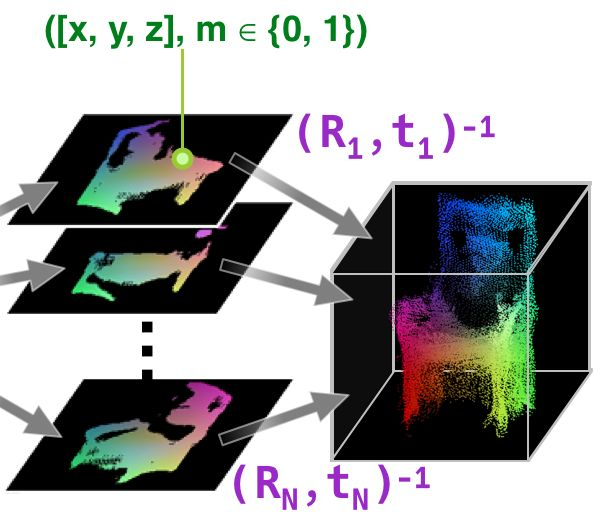• 输入：预先设定视角的 2D 投影

• 输出：点云。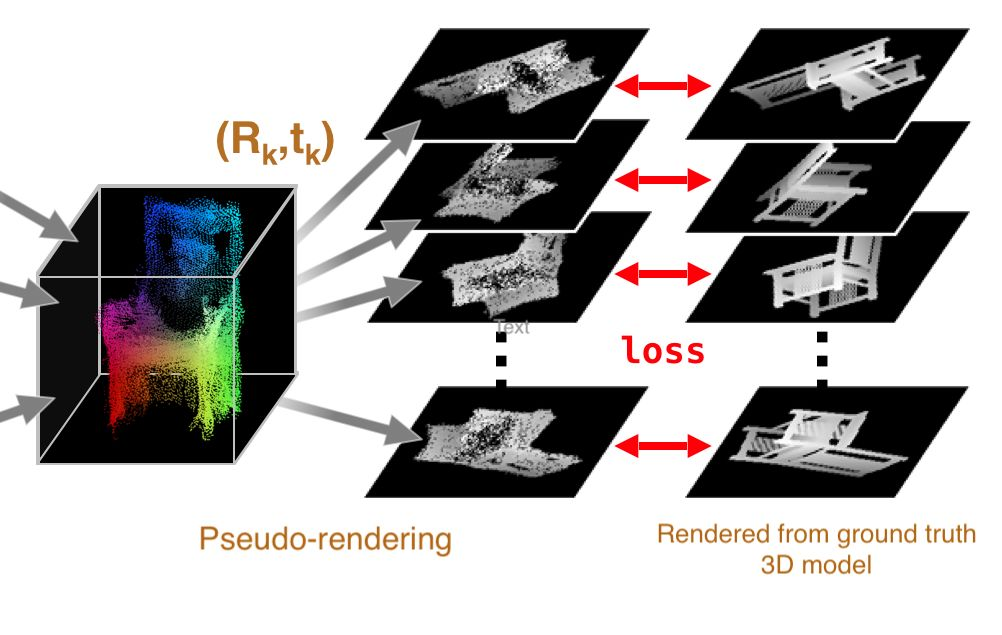• 输入：点云

• 输出：新视角的深度图像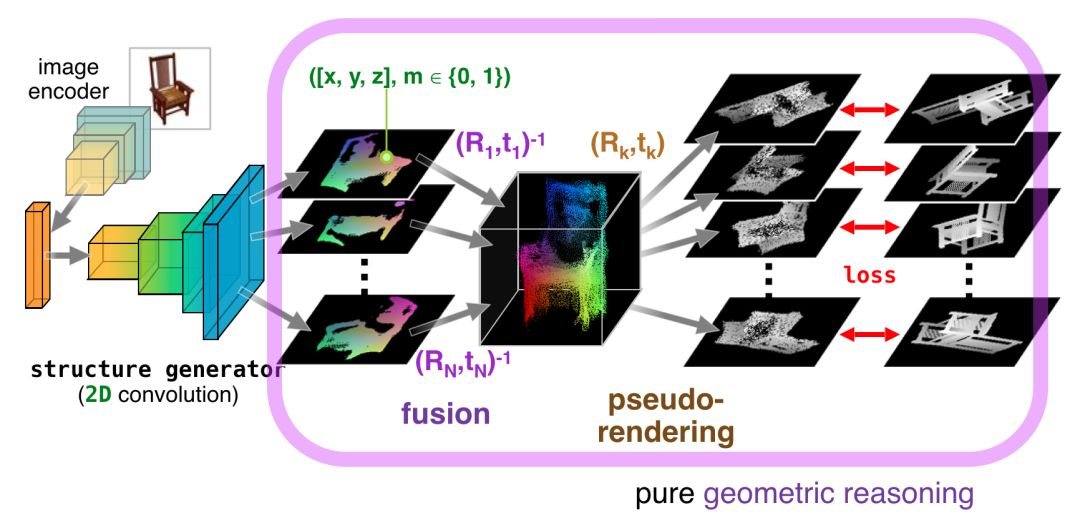• 几何代数意味着没有可学习的参数，这使得模型更小、更易于训练。

• 可微分意味着我们可以反向传播梯度，从而用 2D 投影的损失来学习生成 3D 点云。

``````# --------- Pytorch pseudo-code for training loop ----------#
# Create 2D Conv Structure generator
model = Structure_Generator()
# only need to learn the 2D structure optimizer
optimizer = optim.SGD(model.parameters())
# 2D projections from predetermined viewpoints
XYZ, maskLogit = model(RGB_images)
# fused point cloud
#fuseTrans is predetermined viewpoints info
XYZid, ML = fuse3D(XYZ, maskLogit, fuseTrans)
# Render new depth images at novel viewpoints
# renderTrans is novel viewpoints info
newDepth, newMaskLogit, collision = render2D(XYZid, ML, renderTrans)
# Compute loss between novel view and ground truth
loss_depth = L1Loss()(newDepth, GTDepth)
loss_mask = BCEWithLogitLoss()(newMaskLogit, GTMask)
loss_total = loss_depth + loss_mask
# Back-propagation to update Structure Generator
loss_total.backward()
optimizer.step()``````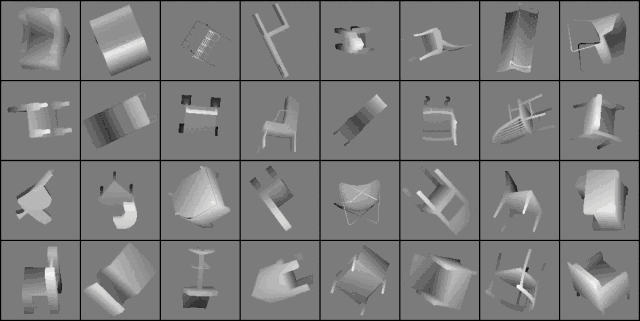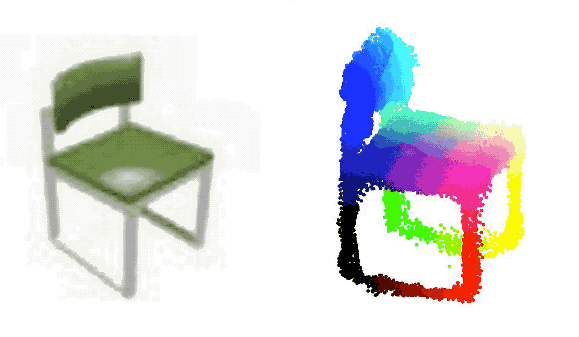• Pytorch 代码：https://github.com/lkhphuc/pytorch-3d-point-cloud-generation

• Tensorflow 代码：https://github.com/chenhsuanlin/3D-point-cloud-generation

• 论文：https://arxiv.org/abs/1706.07036

• 原始项目网站：https://chenhsuanlin.bitbucket.io/3D-point-cloud-generation/